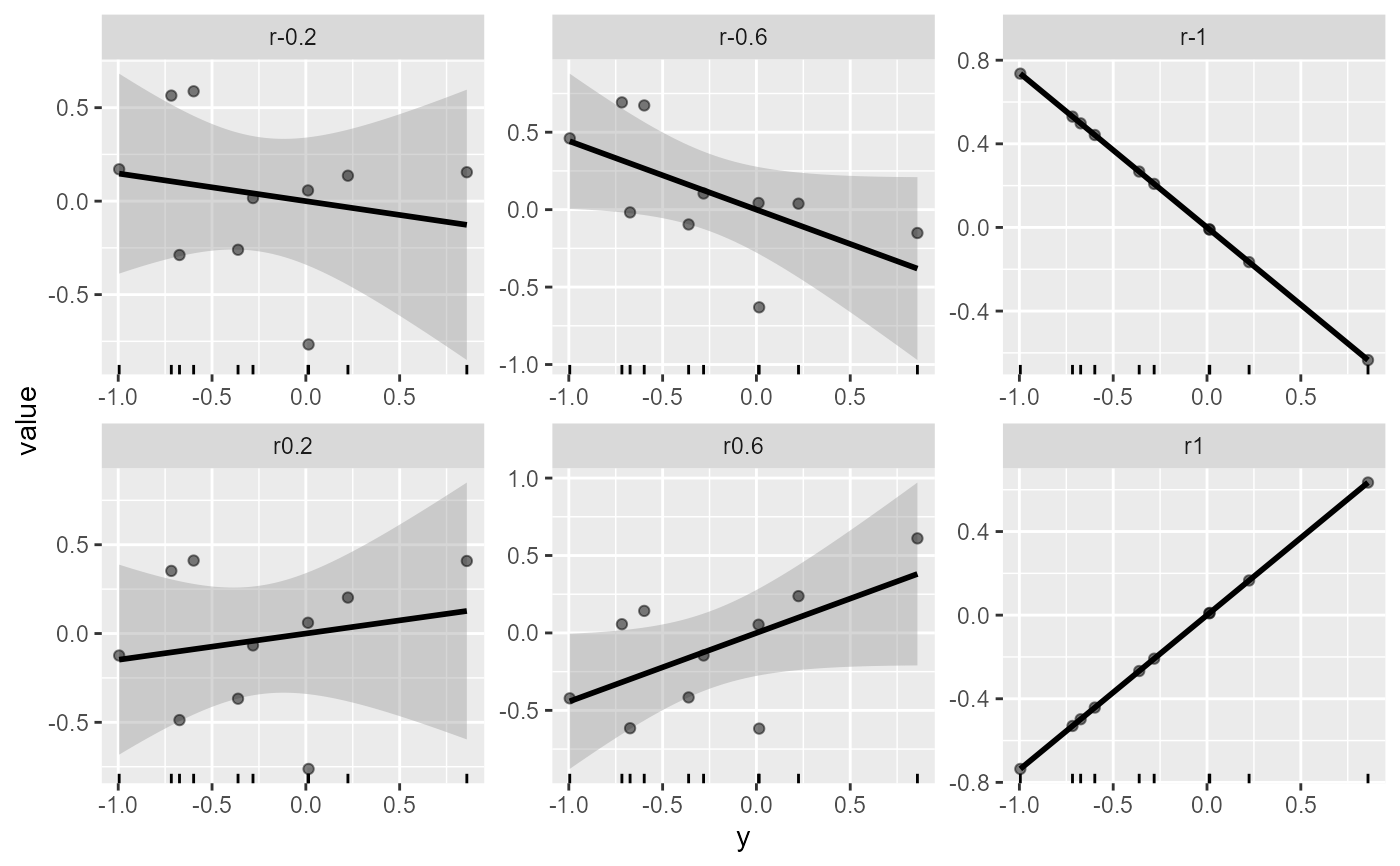Generate correlated variables using a vector of know values and desired maximum and minimum correlations

## Usage

correlated_vars(
y,
min_cor = -1,
max_cor = 1,
nvars,
constant = NULL,
operation = "*",
x = NULL
)

## Arguments

y

A vector to generate variables correlated with.

min_cor

The minimum desired correlation.

max_cor

The maximum desired correlation.

nvars

The number of variables.

constant

A constant. Use operation to define which operation is used.

operation

The operation to be applied to the constant value.

x

An optional vector of the same length of y. If not informed (default) then a normally distributed variable (mean = 0, sd = 1) will be used.

## Value

A data frame with the y variable and the correlated variables.

## Author

Tiago Olivoto tiagoolivoto@gmail.com

## Examples

# \donttest{
library(metan)
y <- rnorm(n = 10)
cor_vars <- correlated_vars(y, nvar = 6)
plot(cor_vars)# }